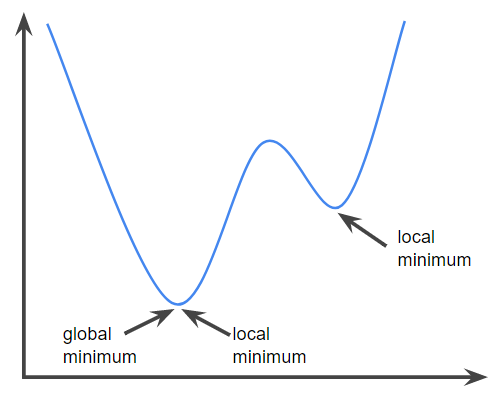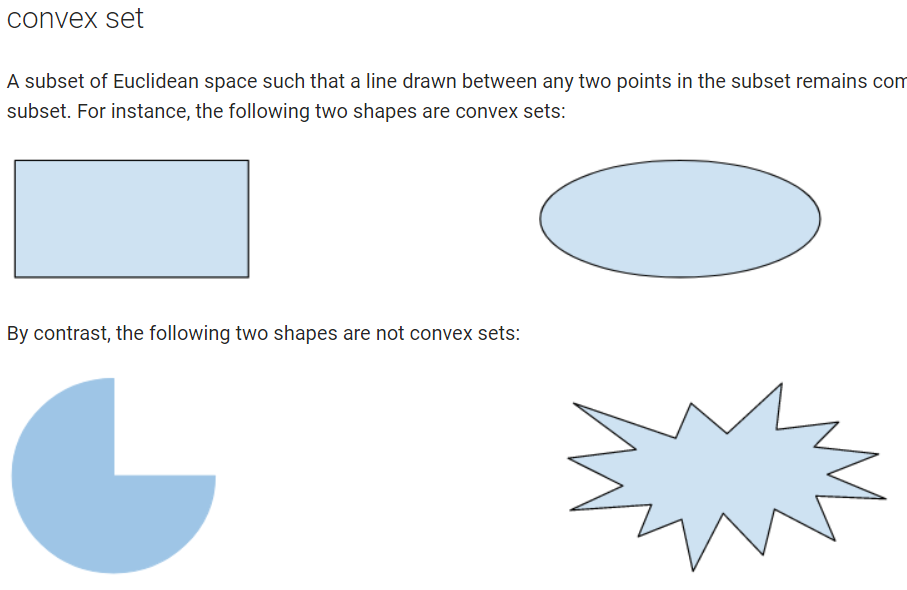#2018 iT 邦幫忙鐵人賽10
AI & Machine Learning

## 前言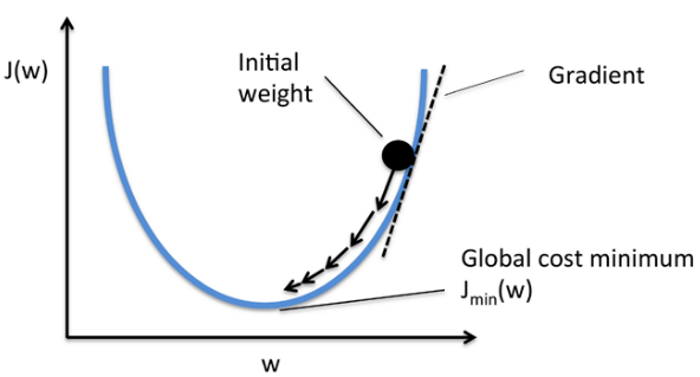## 圖解

Neural Network 的處理過程如下：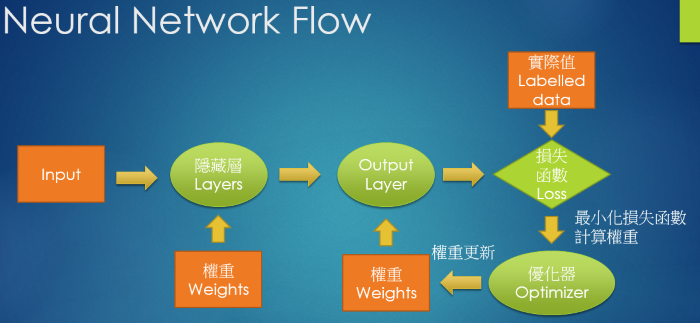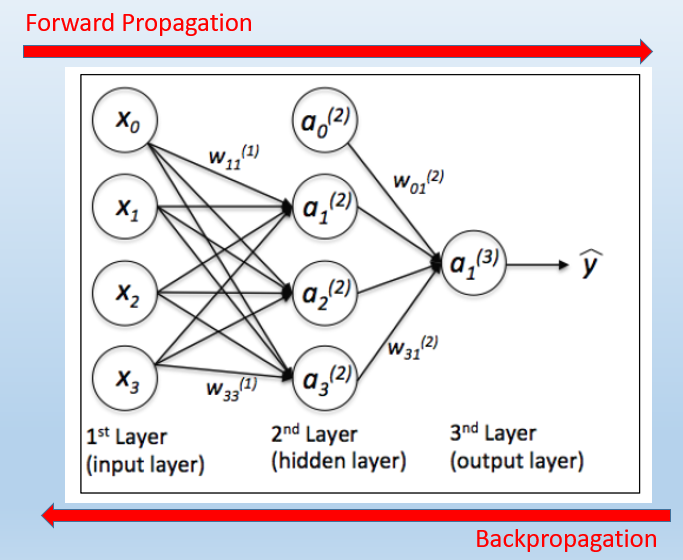1. 先任意假設一組權重(W)，如圖中紅圈內數字。
2. 每一層的 output 就等於 前一層的 input 乘以權重(W)， 即 y = sum(w * x)，這個過程就是 Forward Propagation。舉例來說，隱藏層的第一個節點(Node)就等於 2 * 1 + 3 * 1 = 5, 第二個節點就等於 2 * -1 + 3 * 1 = 1，output層就等於 5 * 2 + 1 * -1 = 9。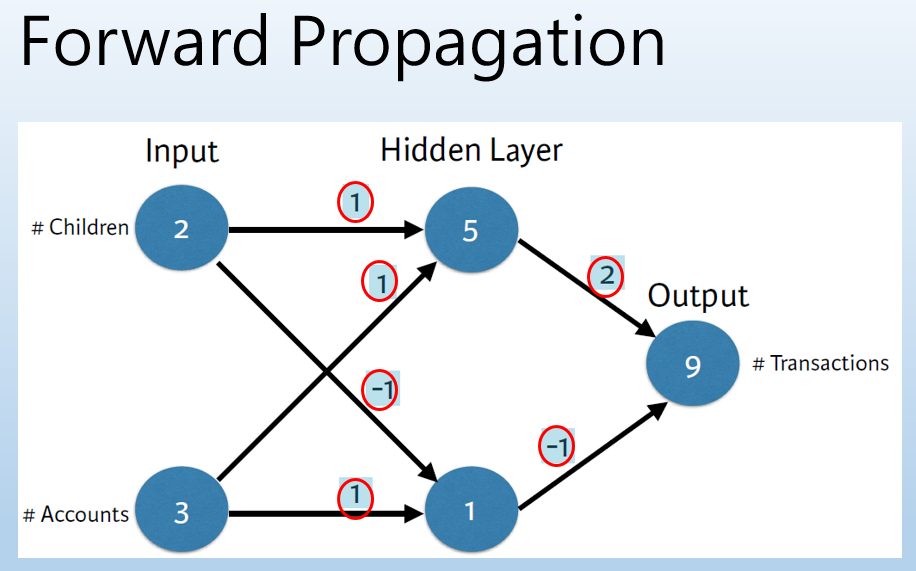1. 接著，我們就反推回去(Backpropagation)，用『梯度下降法』，逐步調整權重(W)，慢慢逼近最佳解，以達到『損失函數最小化』。如圖一，我們先求算梯度(Gradient)，再依梯度往下走，損失就會越來越小，梯度的公式等於 -2 * input * (y實際值 - y 預測值)，後續會證明。再接續上圖，假設已知資料的ouput實際值為 13，那損失就等於 13-9 = 4，這時的output對隱藏層兩個節點的梯度就分別等於 -2 * 5 * 4 = -40 及 -2 * 1 * 4 = -8，因此，我們，就可以設定下降的步幅，即學習率(Learning Rate)，譬如 0.01，所以，調整新權重 = 原權重 - (學習率 * 梯度)，推算出所有的權重後，再回到步驟2，不斷循環，直到損失的縮小已經不顯著了，我們就認定那一組權重是最佳解了。公式整理如下：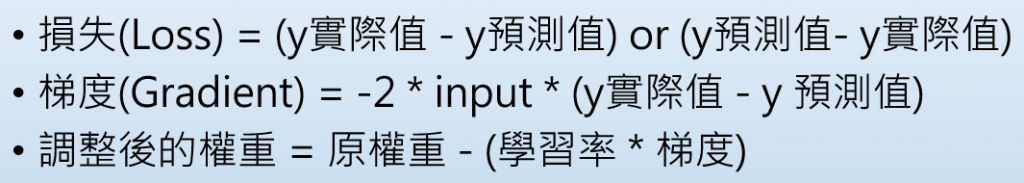## 梯度數學證明• 如果有 bias：即 y = b + w * x，只要等式兩邊各減 b，變成 y' = w * x，還是可以用上圖推導，公式不變。
• 通常會看到 SSE 會乘上 ½，書上說是為了計算方便。

## 範例程式

``````import numpy as np
import matplotlib.pyplot as plt

# 目標函數:y=x^2
def func(x): return np.square(x)

# 目標函數一階導數:dy/dx=2*x
def dfunc(x): return 2 * x

def GD(x_start, df, epochs, lr):
"""  梯度下降法。給定起始點與目標函數的一階導函數，求在epochs次反覆運算中x的更新值
:param x_start: x的起始點
:param df: 目標函數的一階導函數
:param epochs: 反覆運算週期
:param lr: 學習率
:return: x在每次反覆運算後的位置（包括起始點），長度為epochs+1
"""
xs = np.zeros(epochs+1)
x = x_start
xs = x
for i in range(epochs):
dx = df(x)
# v表示x要改變的幅度
v = - dx * lr
x += v
xs[i+1] = x
return xs

# Main
# 起始權重
x_start = 5
# 執行週期數
epochs = 15
# 學習率
lr = 0.3
# 梯度下降法
x = GD(x_start, dfunc, epochs, lr=lr)
print (x)
# 輸出：[-5.     -2.     -0.8    -0.32   -0.128  -0.0512]

color = 'r'
#plt.plot(line_x, line_y, c='b')
from numpy import arange
t = arange(-6.0, 6.0, 0.01)
plt.plot(t, func(t), c='b')
plt.plot(x, func(x), c=color, label='lr={}'.format(lr))
plt.scatter(x, func(x), c=color, )
plt.legend()

plt.show()

``````
• 方式執行： python gd1.py

• 結果如下圖，藍色曲線是損失函數或目標函數，紅色折線是以『梯度下降法』逼近最佳解的過程(由上而下)。

• dfunc 是 func 偏微分的公式，X^2 偏微分等於 2 * X，讀者可以同時改變 func、dfunc 內容，試試看結果是否依然正確。

• 調整其它 Hyperparameters，例如 x_start、epochs、lr，測試逼近的過程。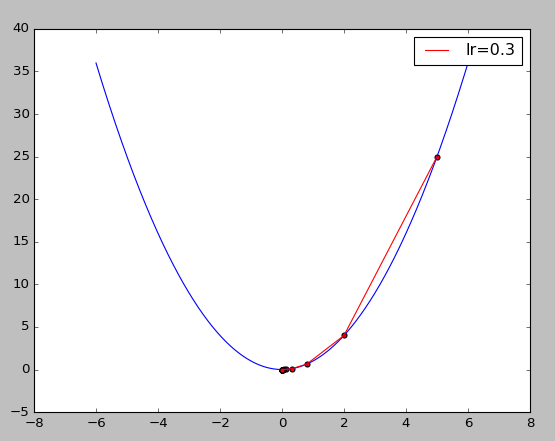• 學習率(lr) 設的過大就會像下圖右，左右跳動，可能求不到最佳解。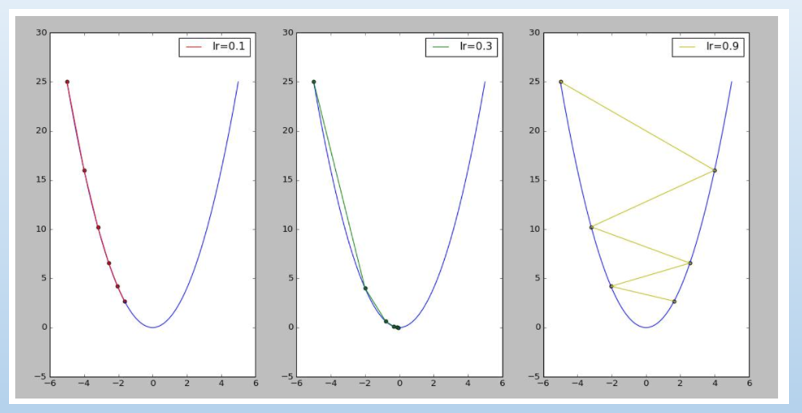圖片來源：『梯度下降法快速教程 _ 第一章：Python简易实现以及对学习率的探讨 - CSDN博客』

## 結語

### 1 則留言

2